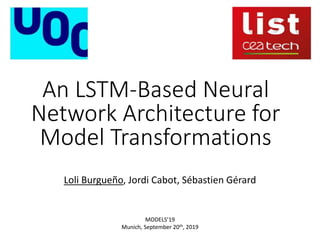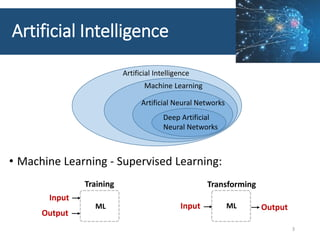Se está descargando tu SlideShare. ×

# An LSTM-Based Neural Network Architecture for Model Transformations

Anuncio
Anuncio
Anuncio
Anuncio
Anuncio
Anuncio
Anuncio
Anuncio
Anuncio
Anuncio
AnuncioCargando en…3
×

1 de 17 Anuncio

# An LSTM-Based Neural Network Architecture for Model Transformations

We propose to take advantage of the advances in Artificial Intelligence and, in particular, Long Short-Term Memory Neural Networks (LSTM), to automatically infer model transformations from sets of input-output model pairs.

We propose to take advantage of the advances in Artificial Intelligence and, in particular, Long Short-Term Memory Neural Networks (LSTM), to automatically infer model transformations from sets of input-output model pairs.

Anuncio
Anuncio

### An LSTM-Based Neural Network Architecture for Model Transformations

1. 1. An LSTM-Based Neural Network Architecture for Model Transformations Loli Burgueño, Jordi Cabot, Sébastien Gérard MODELS’19 Munich, September 20th, 2019
2. 2. 2
3. 3. Artificial Intelligence • Machine Learning - Supervised Learning: 3 Input Output Training Transforming ML Input OutputML Artificial Intelligence Machine Learning Artificial Neural Networks Deep Artificial Neural Networks
4. 4. Artificial Neural Networks • Graph structure: Neurons + directed weighted connections • Neurons are mathematical functions • Connections are weights • Adjusted during the learning process to increase/decrease the strength of the connection 4
5. 5. Artificial Neural Networks • The learning process basically means to find the right weights • Supervised learning methods. Training phase: • Example input-output pairs are used (Dataset) 5 Dataset Training Validation Test
6. 6. Artificial Neural Networks • Combine two LSTM for better results • Avoids fixed size input and output constraints 6 • MTs ≈ sequence-to-sequence arch
7. 7. Architecture • Encoder-decoder architecture + • Long short-term memory neural networks 7 Encoder LSTM network Decoder LSTM network InputModel OutputModel
8. 8. Architecture • Sequence-to-Sequence transformations • Tree-to-tree transformations • Input layer to embed the input tree to a numeric vector + • Output layer to obtain the output model from the numeric vectors produced by the decoder 8 InputTree EmbeddingLayer Encoder LSTM network OutputTree ExtractionLayer Decoder LSTM network InputModel OutputModel
9. 9. • Attention mechanism • To pay more attention (remember better) to specific parts • It automatically detects to which parts are more important 9 Architecture InputTree EmbeddingLayer Encoder LSTM network OutputTree ExtractionLayer Decoder LSTM network AttentionLayer InputModel OutputModel
10. 10. • Pre- and post-processing required to… • represent models as trees • reduce the size of the training dataset by using a canonical form • rename variables to avoid the “dictionary problem” 10 Model pre- and post-processing InputModel (preprocessed) InputTree EmbeddingLayer Encoder LSTM network OutputTree ExtractionLayer OutputModel (non-postprocessed) Decoder LSTM network AttentionLayer InputModel OutputModel Preprocessing Postprocessing
11. 11. Preliminary results Class to Relational case study 11
12. 12. Model representation 12 MODEL ASSOCOBJ c Class ATTS isAbstract name false family OBJ a Attribute ATTS multivalued name false surname OBJ dt Datatype ATTS name String att c a ASSOC type a dt
13. 13. Preliminary results Correctness • Measured through the accuracy and validation loss 13
14. 14. Preliminary results 14 Performance 1. How long does it take for the training phase to complete?
15. 15. Preliminary results Performance 1. How long does it take for the training phase to complete? 15 2. How long it takes to transform an input model when the network is trained?
16. 16. Limitations/Discussion • Size of the training dataset • Diversity in the training set • Computational limitations of ANNs • i.e., mathematical operations • Generalization problem • predicting output solutions for input models very different from the training distribution it has learn from • Social acceptance 16
17. 17. An LSTM-Based Neural Network Architecture for Model Transformations Loli Burgueño, Jordi Cabot, Sébastien Gérard MODELS’19 Munich, September 20th, 2019

### Notas del editor

• We were inspired by natural language translation and thought, why don’t we try to translate/transform models?
• The correctness of ANNs is studied through its accuracy and overfitting (being the latter measured through the validation loss). The accuracy should be as close as 1 as possible while the validation loss as close to 0 as possible.

The accuracy is calculated comparing for each input model in the test dataset whether the output of the network corresponds with the expected output. If it does, the network was able to successfully predict the target model for the given input model.

The accuracy grows and the loss decreases with the size of the dataset, i.e., the more input-output pairs we provide for training, the better our software learns and predicts (transforms). In this concrete case, with a dataset with 1000 models, the accuracy is 1 and the loss 0 (meaning that no overfitting was taking place), which means that the ANNs are perfectly trained and ready to use. Note that we show the size of the complete dataset but, we have split it using an 80% of the pairs for training, a 10% for validation and another 10% for testing.
• The correctness of ANNs is studied through its accuracy and overfitting (being the latter measured through the validation loss). The accuracy should be as close as 1 as possible while the validation loss as close to 0 as possible.

The accuracy is calculated comparing for each input model in the test dataset whether the output of the network corresponds with the expected output. If it does, the network was able to successfully predict the target model for the given input model.

The accuracy grows and the loss decreases with the size of the dataset, i.e., the more input-output pairs we provide for training, the better our software learns and predicts (transforms). In this concrete case, with a dataset with 1000 models, the accuracy is 1 and the loss 0 (meaning that no overfitting was taking place), which means that the ANNs are perfectly trained and ready to use. Note that we show the size of the complete dataset but, we have split it using an 80% of the pairs for training, a 10% for validation and another 10% for testing.